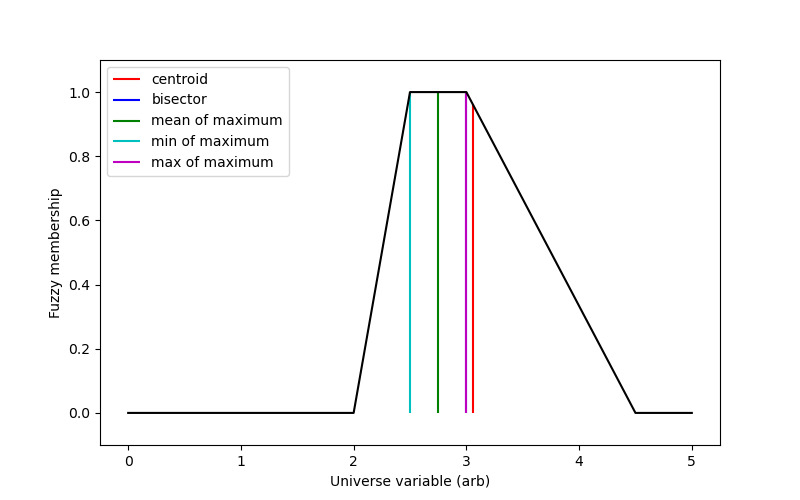# Defuzzification¶

Fuzzy logic calculations are excellent tools, but to use them the fuzzy result must be converted back into a single number. This is known as defuzzification.

There are several possible methods for defuzzification, exposed via skfuzzy.defuzz.```import numpy as np
import matplotlib.pyplot as plt
import skfuzzy as fuzz

# Generate trapezoidal membership function on range [0, 1]
x = np.arange(0, 5.05, 0.1)
mfx = fuzz.trapmf(x, [2, 2.5, 3, 4.5])

# Defuzzify this membership function five ways
defuzz_centroid = fuzz.defuzz(x, mfx, 'centroid')  # Same as skfuzzy.centroid
defuzz_bisector = fuzz.defuzz(x, mfx, 'bisector')
defuzz_mom = fuzz.defuzz(x, mfx, 'mom')
defuzz_som = fuzz.defuzz(x, mfx, 'som')
defuzz_lom = fuzz.defuzz(x, mfx, 'lom')

# Collect info for vertical lines
labels = ['centroid', 'bisector', 'mean of maximum', 'min of maximum',
'max of maximum']
xvals = [defuzz_centroid,
defuzz_bisector,
defuzz_mom,
defuzz_som,
defuzz_lom]
colors = ['r', 'b', 'g', 'c', 'm']
ymax = [fuzz.interp_membership(x, mfx, i) for i in xvals]

# Display and compare defuzzification results against membership function
plt.figure(figsize=(8, 5))

plt.plot(x, mfx, 'k')
for xv, y, label, color in zip(xvals, ymax, labels, colors):
plt.vlines(xv, 0, y, label=label, color=color)
plt.ylabel('Fuzzy membership')
plt.xlabel('Universe variable (arb)')
plt.ylim(-0.1, 1.1)
plt.legend(loc=2)

plt.show()
```

Python source code: `download` (generated using `skimage` 0.4.2)

IPython Notebook: `download` (generated using `skimage` 0.4.2)